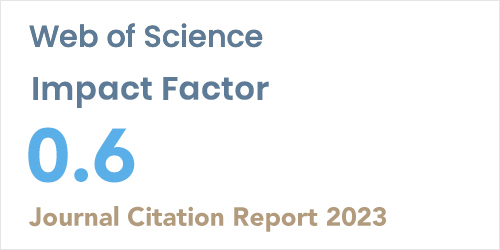ISSN: 1304-7191 | E-ISSN: 1304-7205
Volume: 41 Issue: 4 Year: 2023Genocchi polynomial method for the multiterm variable-order fractional differential equations
1Department of Electronics and Communications Engineering, Yıldız Technical University, Istanbul, Turkey
2Department of , Mathematical Engineering, Yıldız Technical University, Istanbul, Turkey
Sigma J Eng Nat Sci 2022; 40(1): 79-84 DOI: 10.14744/sigma.2021.00032

#### Abstract

In this paper a numerical solution for multiterm varable-order fractional differential equations (VO-FDEs) using Genocchi polynomials is proffered. By making use of the Genocchi polynomials, a multiterm VO-FDE can be approximated by a corresponding system of algebraic equations. To be able to do that, operational matrices for variable order fractional differentials are obtained using Genocchi polynomials. Then the algebraic equation system is solved for the coefficient values, thus the approximate solution is obtained by using the linear combination of those coefficients. Numerical examples are provided.